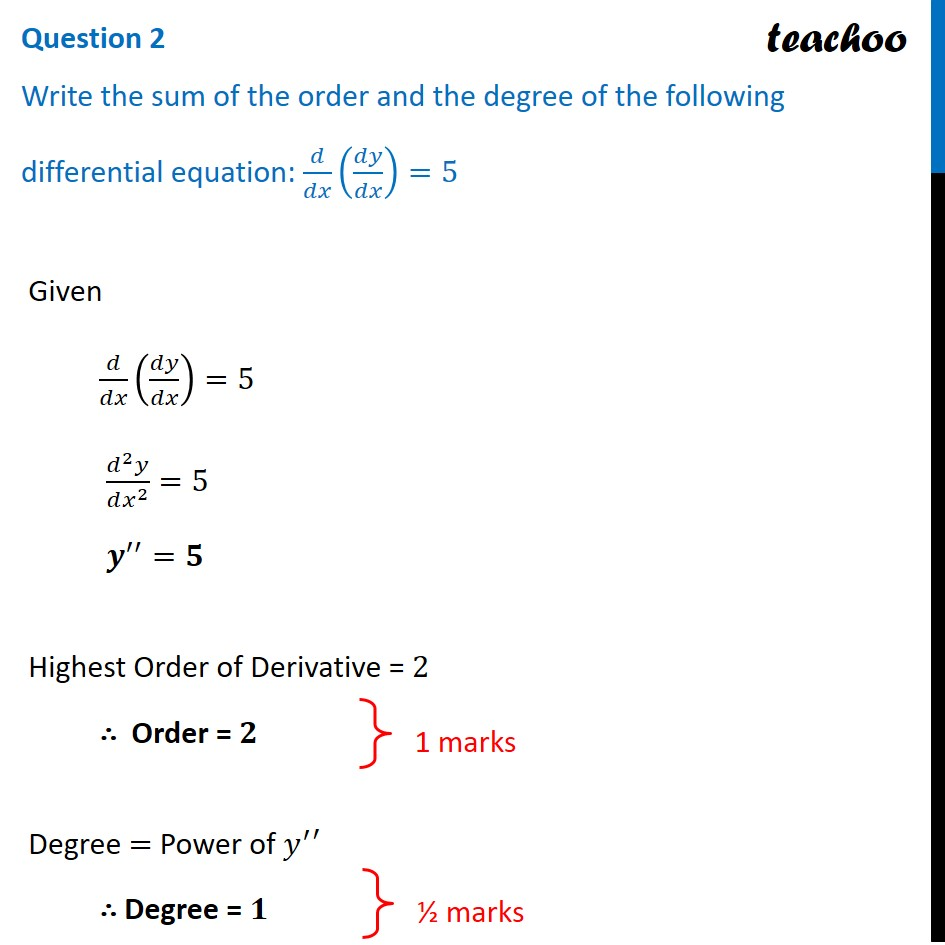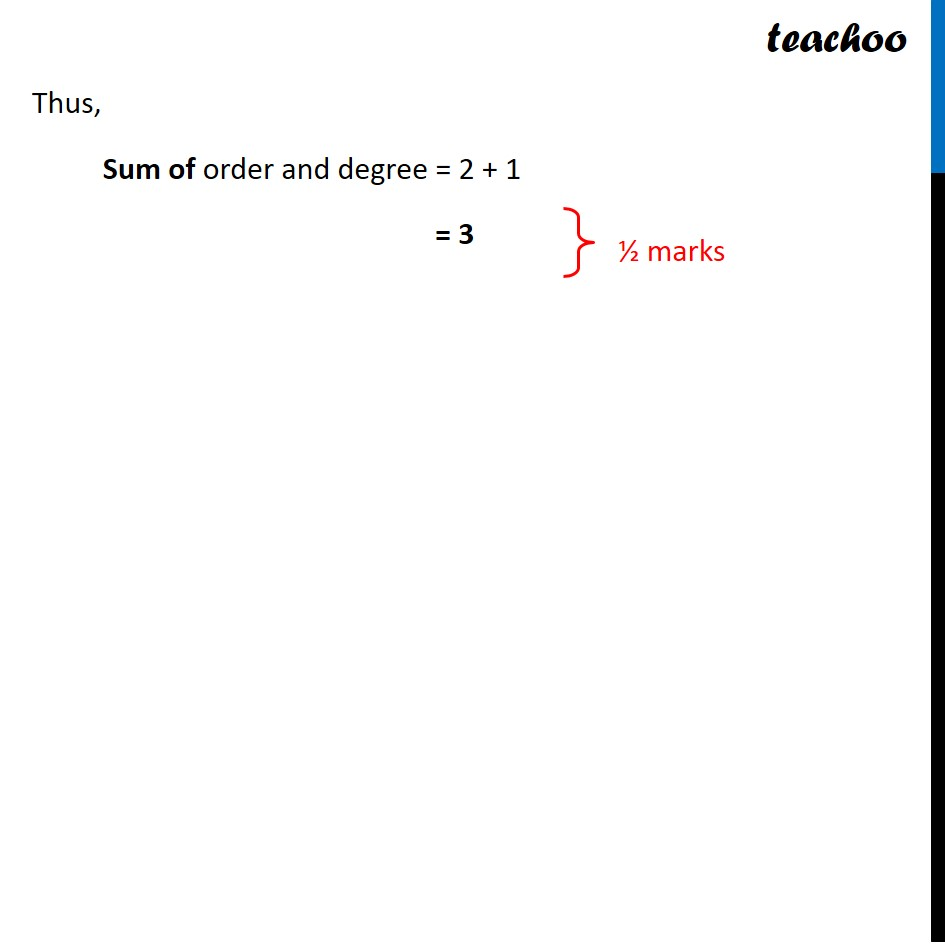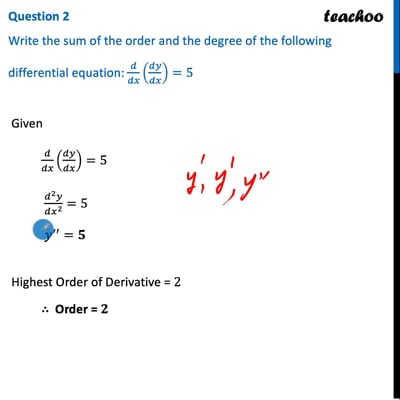CBSE Class 12 Sample Paper for 2022 Boards (For Term 2)

Class 12
Solutions of Sample Papers and Past Year Papers - for Class 12 Boards

## Write the sum of the order and the degree of the following differential equation  : d/dx (dy/dx) = 5This video is only available for Teachoo black users

Learn in your speed, with individual attention - Teachoo Maths 1-on-1 Class

### Transcript

Question 2 Write the sum of the order and the degree of the following differential equation: 𝑑/𝑑𝑥 (𝑑𝑦/𝑑𝑥)=5Given 𝑑/𝑑𝑥 (𝑑𝑦/𝑑𝑥)=5 (𝑑^2 𝑦)/(𝑑𝑥^2 )=5 𝒚^′′=𝟓 Highest Order of Derivative = 2 ∴ Order = 𝟐 Degree = Power of 𝑦^′′ ∴ Degree = 𝟏 Thus, Sum of order and degree = 2 + 1 = 3 ½ marks